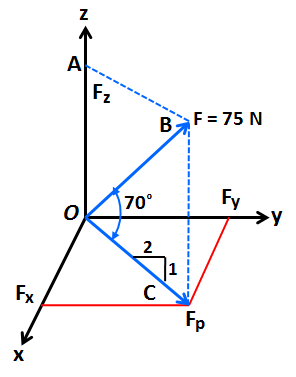# (3) Express the 75'' N'' force as a vector and give its direction cosines. Also, express the...

## Question:

(3) Express the 75 N force as a vector and give its direction cosines. Also, express the direction in a unit vector form.## Vector

A vector has both magnitude and direction as opposed to a scalar quantity which has a magnitude but no direction. For example, speed is a scalar quantity which only has a magnitude like 40 m/s. But velocity is a vector which has magnitude and direction like 40 m/s along X-axis.

From the figure,

{eq}\displaystyle F_z = F* sin70° \\ F_z = 75*0.9397 = 70.477 \ N {/eq}

{eq}\displaystyle F_p = F*cos70° \\ F_p = 75*0.342 = 25.651 \ N {/eq}

From the given slope, the angle made by {eq}F_p {/eq} with the Y-axis is,

{eq}\displaystyle \beta = tan^{-1} ( \frac {1}{2} ) \\ \beta = 26.565° {/eq}

Therefore,

{eq}F_x = F_p*sin 26.565° = 11.471 \ N \\ F_y = F_p*cos 26.565° = 22.943 \ N {/eq}

The force of 75N, expressed in vector form is {eq}11.471i + 22.943j + 70.477k {/eq}

The direction cosines are,

{eq}\displaystyle cos \alpha = \frac {11.471}{75} = 0.153 \\ cos \beta = \dfrac{22.943}{75} = 0.306 \\ cos \gamma = \dfrac{70.477}{75} = 0.940 \\ {/eq}

The unit vector is expressed as,

{eq}\displaystyle cos \alpha i + cos \beta j + cos \gamma j \\ 0.153 i + 0.306 j + 0.940 k {/eq}